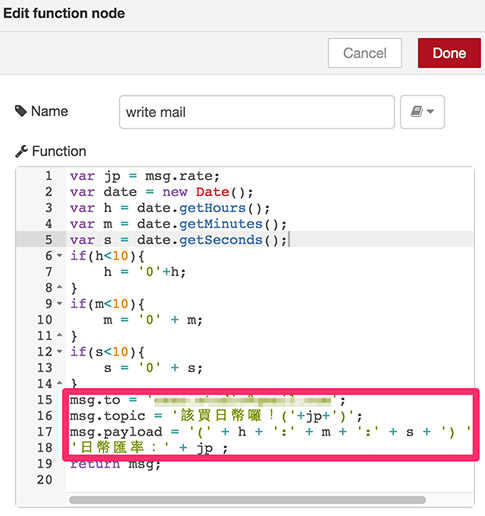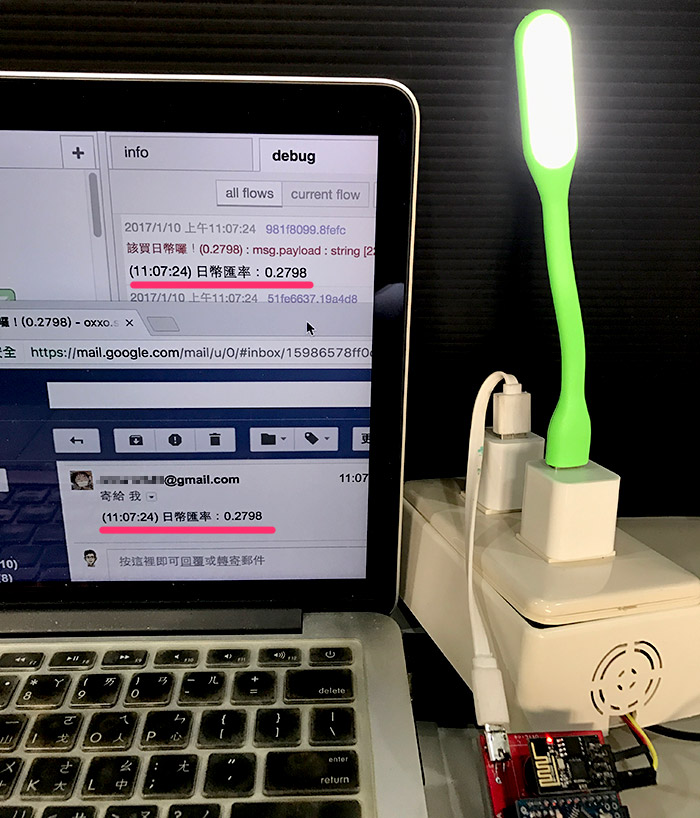Webduino 學習手冊網站即將改版，提供您更好的閱讀體驗！

# 日幣匯率 ( Node-Red 爬蟲 )

## 解析匯率網頁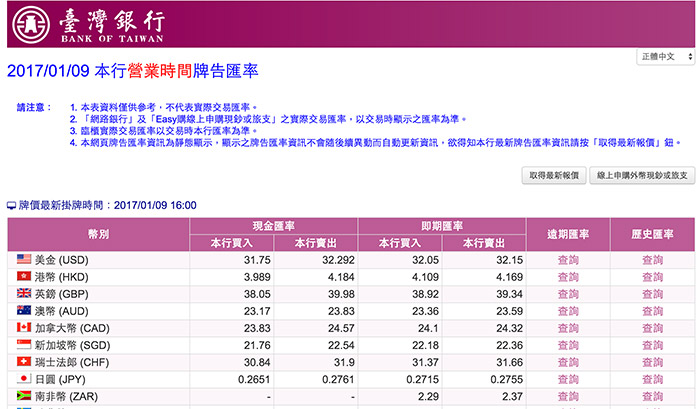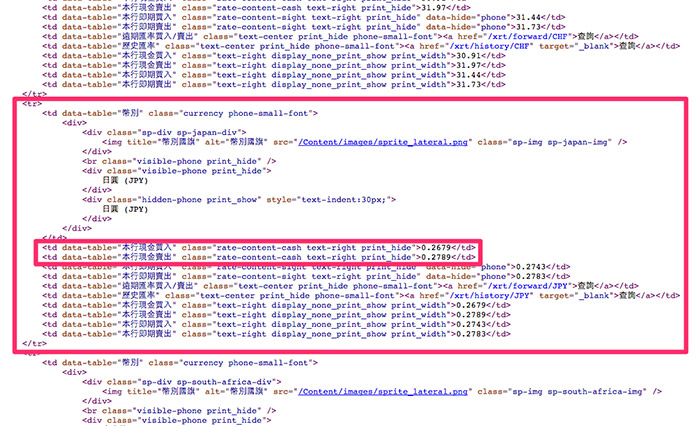``````rate-content-cash text-right print_hide
``````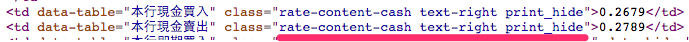## 使用 Node-RED 抓取匯率數值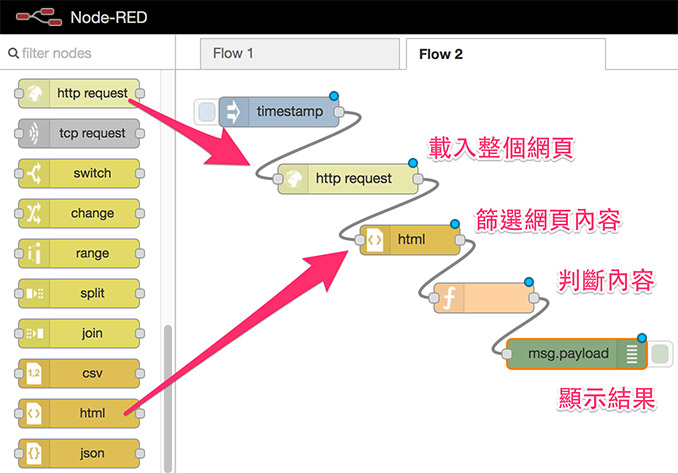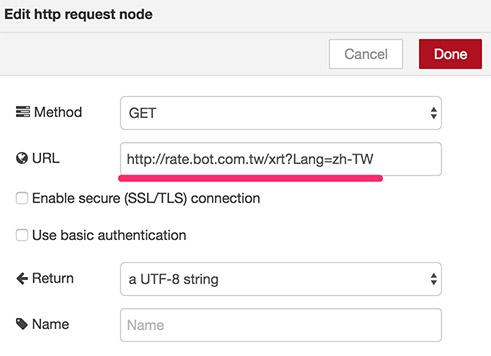Output 選擇 `the html content``only the text content` 都可以，設定好之後可以賦予這個標籤一個名稱，我這邊給它命名為 filter。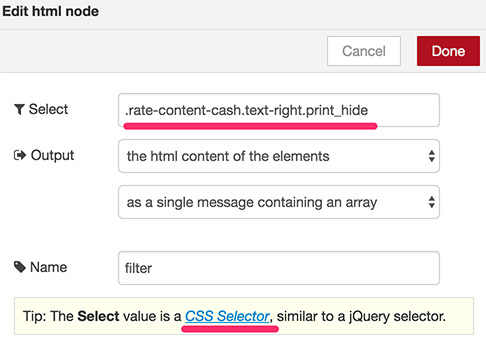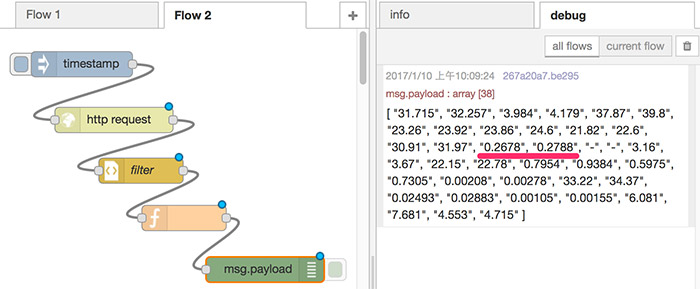``````var jp = msg.payload;
var date = new Date();
var h = date.getHours();
var m = date.getMinutes();
var s = date.getSeconds();
if(h<10){
h = '0'+h;
}
if(m<10){
m = '0' + m;
}
if(s<10){
s = '0' + s;
}
msg.payload = '(' + h + ':' + m + ':' + s + ')\n'+
'日幣匯率：' + jp ;
return msg;
``````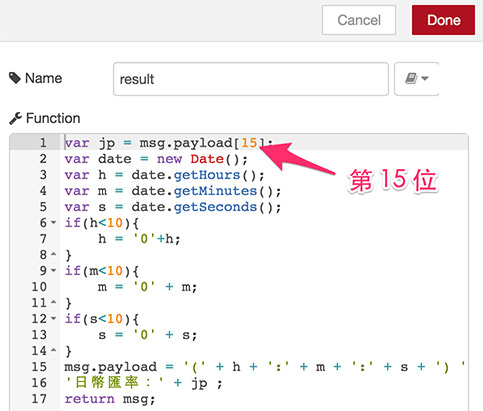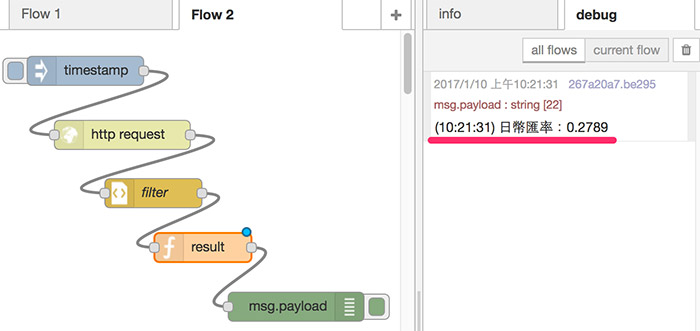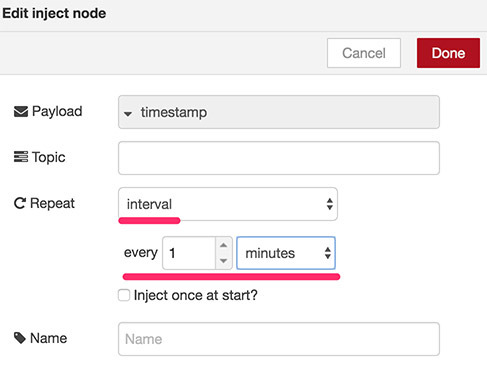## 到達匯率時，寄送 Email 並透過 Webduino 點亮燈泡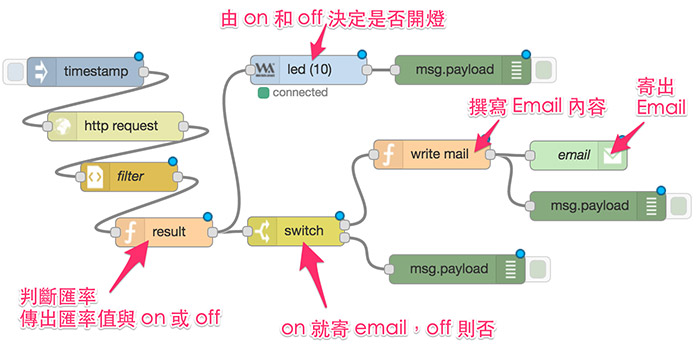``````var jp = msg.payload;
msg.rate = jp;
if(jp<0.29){
}else{
}
return msg;
``````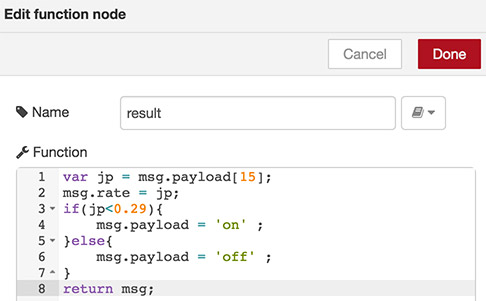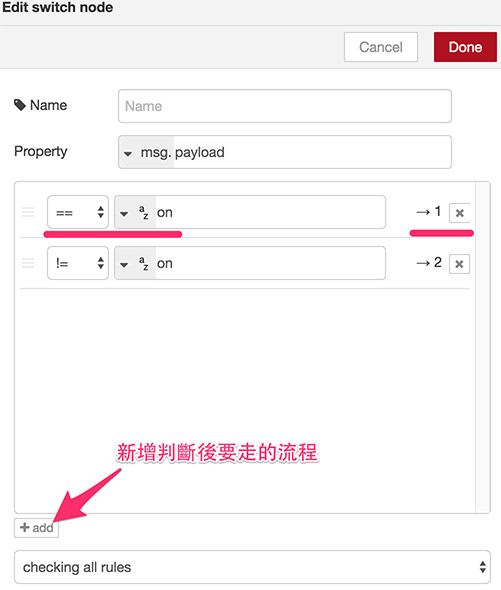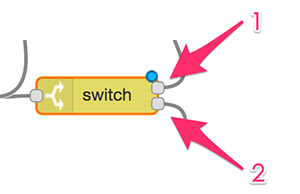``````var jp = msg.rate;
var date = new Date();
var h = date.getHours();
var m = date.getMinutes();
var s = date.getSeconds();
if(h<10){
h = '0'+h;
}
if(m<10){
m = '0' + m;
}
if(s<10){
s = '0' + s;
}
msg.to = '[email protected]';
msg.topic = '該買日幣囉！('+jp+')';
msg.payload = '(' + h + ':' + m + ':' + s + ') '+
'日幣匯率：' + jp ;
return msg;
``````